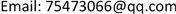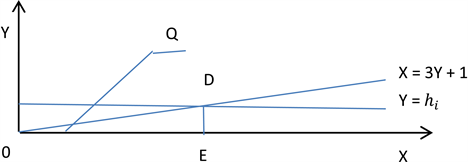﻿ 证明角谷猜想是正确的 Proof of Collatz Conjecture

Pure Mathematics
Vol. 09  No. 03 ( 2019 ), Article ID: 30362 , 3 pages
10.12677/PM.2019.93055

Proof of Collatz Conjecture

Sanzhong Zou

Guangzhou GuangdongReceived: Apr. 26th, 2019; accepted: May 6th, 2019; published: May 22nd, 2019ABSTRACT

Assume that Collatz conjecture is incorrect, and represent the set of natural numbers as N, then the N set can be divided into B set and H set. Set B satisfies the Collatz conjecture; set H does not satisfy the Collatz conjecture. After performing the Collatz operation on the number in the H, it proves that the Collatz conjecture is correct.

Keywords:Collatz Algorithm, B Set, H Set1. 预备工作

1) 满足角谷猜想的自然数，记为集合B

$\text{B}=\left\{{b}_{1},{b}_{2},{b}_{3},{b}_{4},\cdots ,{b}_{i}\right\}$,${b}_{1}=1,{b}_{2}=2,{b}_{3}=3,{b}_{4}=4,{b}_{i}\to \infty$

2) 不满足角谷猜想的自然数称为非角谷数集，记为集合H

$\text{H}=\left\{{h}_{1},{h}_{2},{h}_{3},{h}_{4},\cdots ,{h}_{i}\right\}$

2. 命题证明Figure 1. Line $X=3Y+1$ intersects Line $Y={h}_{i}$

${h}_{i+1}=\frac{{\left(3{h}_{1}+1\right)}^{i}}{{h}_{1}^{i-1}{2}^{{n}_{1}+{n}_{2}+\cdots +{n}_{i}}}$,$\left({n}_{1}+{n}_{2}+\cdots +{n}_{i}\right)\ge i$ , (1)

${n}_{1}={n}_{2}=\cdots ={n}_{i}=1$ 时， $\left({n}_{1}+{n}_{2}+\cdots +{n}_{i}\right)=i$，设 $\left({n}_{1}+{n}_{2}+\cdots +{n}_{i}\right)=\beta ,\beta \ge i$

Proof of Collatz Conjecture[J]. 理论数学, 2019, 09(03): 414-416. https://doi.org/10.12677/PM.2019.93055

1. 1. Gu, C.H. (1992) Mathematics Dictionary. Shanghai Dictionary Press, Shanghai.

2. 2. Min, S.H. (1981) Method of Number Theory. Science Press, Beijing.

3. NOTES

作者简介：出生年月：1959年9月，籍贯：广东省始兴县，学历：本科，职称：工程师。